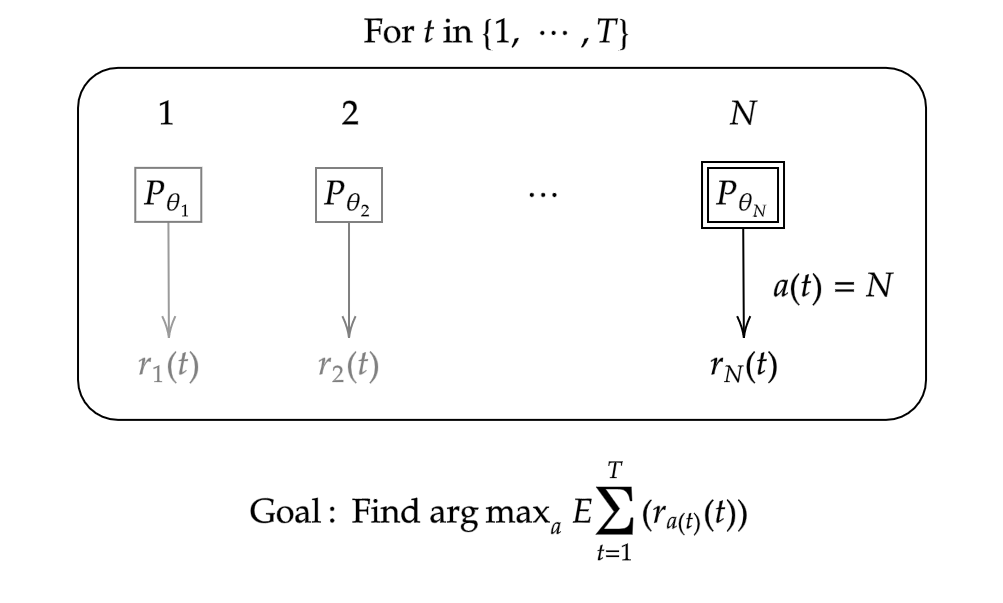# 1. Introduction to Multi-armed Bandit

$\newcommand{\argmin}{\mathop{\mathrm{argmin}}\limits}$ $\newcommand{\argmax}{\mathop{\mathrm{argmax}}\limits}$

The [Bandit] series of posts is my memo on the lecture Seminar in Recent Development of Applied Statistics (Spring, 2021) by Prof. Myunghee Cho Paik. This lecture focuses on adaptive sequential decision making. To be more specific, it covers wide variants of bandit problems.

The first course is a beginner’s guide to the problem settings.

## The bandit problem

Adative sequential decision making is a family of problems and solutions where the data becomes available in a sequential order as time $t$ progresses. The algorithm (sometimes referred to as player or user) choose the action at time $t$ given the data in hand, which makes it adaptive. Each time the algorithm selects an action, the reward associated to that action is revealed.

Our goal is to build an algorithm that maximizes the expected cumulative rewards, under uncertainty. In other words, we want to identify the best action and choose it by observing rewards (with intrinsic randomness) of our choice at each time step. The problem can be viewed as a special case of reinforcement learning and online learning.

### Multi-armed bandit

The problem discussed in the above can be described by multiple one-armed bandits, or multi-armed bandit (MAB). Condiser a row of $N$ different slot machines (also known as one-armed bandits). Each bandit, denoted as $i\in\{1,\cdots,N\}$, has probability distribution $P_{\theta_i(t)}$ parametrized by $\theta_i(t)$ that generates random rewards $r_i(t)$ at each time step $t.$ A bandit algorithm choose one of the possible arms (or actions, or bandits) $a(t)$ at each time point $t$. Our goal is to find a bandit $a$ that finds and chooses the arms in each step that maximizes the total expected rewards.Fig 1. Diagram of multi-armed bandit problem. Only the reward from the chosen arm (double-bordered) is revealed.

Unlike standard supervised learning settings, only the reward from the chosen arm is revealed. Some variants of multi-armed bandit consider a setting where the rewards from not only the chosen but some rejected arms are also revealed (partial information). In order to identify the best arm, we need to explore for possible best one while also exploit the identified one.

## Assumptions on rewards

Variants of multi-armed bandits assumes one of the followings on rewards.

1. IID rewards. Rewards in each arms are independent and identically distributed.
2. Adversarial rewards. Rewards can be arbitrary. This assumes some rewards as attacks to fool the algorithm.
3. Stochastic rewards. Rewards are independent over time $t.$

## External context

Fixing an arm as the best choice in all conditions might not perform well in real world situations. Observing some external context and choosing the action accordingly might be helpful. Bandit algorithms that takes context into account are called contextual bandits.

While it is similar to reinforcement learning, the difference lies in the nature of context. If contexts does not depend on the previous actions, contextual bandits are suitable. Reinforcement learning is utilized when current context depends on past actions.

References

• Lattimore, Szepesvari. 2020. Bandit Algorithms. Cambridge University .
• Seminar in Recent Development of Applied Statistics (Spring, 2021) @ Seoul National University, Republic of Korea (instructor: Prof. Myunghee Cho Paik).